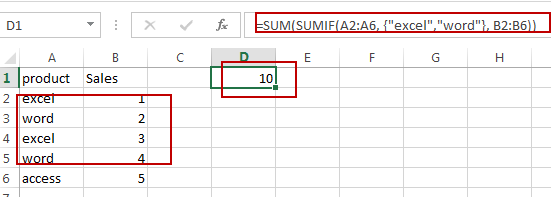# How to Use SUMIF with Multiple Criteria in Same Column in Excel

This post will guide you how to SUMIF with multiple criteria in same column in Excel. How do I use SUMIF with multiple criteria in one column in Excel.

## SUM Cells with Multiple Criteria in Same Column

Assuming that you have a list of data in range A1:B6, in which contain sales data. And you want to sum cells that match two criteria with a formula. The criteria is sum all sales values for both product “excel” and “word”. How to do it. You can use a formula based on the SUM function and the SUMIF function to achieve the result. Like this:

`=SUM(SUMIF(A2:A6, {"excel","word"}, B2:B6))`

Type this formula into a blank cell and press Enter key on your keyboard.You should notice that the total value of matching those two criteria has been calculated successfully.

Note: The range A2:A6 is the range of cells that you want to check the criteria. And {“excel”,”word”} is the criteria, you need to change it as you need.  And the B2:B6 is the cells which you want to sum.

### Related Functions

• Excel SUM function
The Excel SUM function will adds all numbers in a range of cells and returns the sum of these values. You can add individual values, cell references or ranges in excel.The syntax of the SUM function is as below:= SUM(number1,[number2],…)…
• Excel SUMIF Function
The Excel SUMIF function sum the numbers in the range of cells that meet a single criteria that you specify. The syntax of the SUMIF function is as below:=SUMIF (range, criteria, [sum_range])…